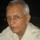Coffee Room
Discuss anything here - everything that you wish to discuss with fellow engineers.
12914 Members
Join this group to post and comment.# cube problem

Imagine a cubic array made up of an n*n*n arrangement of unit cubes: the cubic array is n cubes wide, n cubes high and n cubes deep. A special case is a 3*3*3 Rubik’s cube, which you may be familiar with. How many unit cubes are there on the surface of the n * n * n cubic array?~on*kiyu • Apr 23, 2012
zero~on*kiyu
zeroBanashree Patra
Imagine a cubic array made up of an n*n*n arrangement of unit cubes: the cubic array is n cubes wide, n cubes high and n cubes deep. A special case is a 3*3*3 Rubik’s cube, which you may be familiar with. How many unit cubes are there on the surface of the n * n * n cubic array?
6*n^2 - 12*n + 8 for n > 1silverscorpion • Apr 23, 2012
6n[sup]2[/sup] - 12n + 8

I think it can also be expressed as, n[sup]3[/sup] - (n-2)[sup]3[/sup]silverscorpion
6n[sup]2[/sup] - 12n + 8

I think it can also be expressed as, n[sup]3[/sup] - (n-2)[sup]3[/sup]
The second is more elegant. It represents the actual situation. What we are considering is the outer cube minus the (n-2) cube within it. n^3 is the total number of unit cubes, (n-2)^3 is the number of unit cubes in the contained cube. Aesthetically the second solution is more satisfying.